Courses

# NCERT Exemplar - Structure of the Atom Class 9 Notes | EduRev

## Class 9 : NCERT Exemplar - Structure of the Atom Class 9 Notes | EduRev

The document NCERT Exemplar - Structure of the Atom Class 9 Notes | EduRev is a part of the Class 9 Course Science Class 9.
All you need of Class 9 at this link: Class 9

Q.1. Is it possible for the atom of an element to have one electron, one proton and no neutron? If so, name the element.
Ans
. Yes, the element is hydrogen.

Q.2. Write any two observations which support the fact that atoms are divisible.
Ans.
(a) Discovery of electrons
(b) Discovery of protons
(c) Discovery of neutrons
The existence of these particles show that atoms are divisible.

Q.3.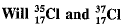have different valencies? Justify your answer.
Ans.
No. They will have the same valencies because their atomic numbers are same, electronic configuration is same and they have the same number of valence electrons.

Q.4. Why did Rutherford select a gold foil in his α-ray scattering experiment?
Ans.
It is because gold is highly malleable and thus a very thin foil of gold can be obtained.

Q.5. Find out the valency of the atoms represented by the figure (a) and (b).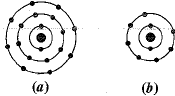Ans.
(a) has valency equal to zero as its octet is complete, i.e. it has 8 electrons in its valence shell.
(b) has valency equal to 1 as it has 7 valence electrons. It can gain or share 1 electron to become stable.

Q.6. One electron is present in the outermost shell of the atom of an element X. What would be the nature and value of charge on the ion formed if this electron is removed f»e outermost shell?
Ans.
It will acquire positive charge and value will be equal to + 1.

Q.7. Write down the electron distribution of chlorine atom. How many electrons are there in the shell? (Atomic number of chlorine is 17).
Ans.
Cl (17) ⇒ K = 2, L = 8, M = 7. The L shell has eight electrons.

Q.8. In the atom of an element X, 6 electrons are present in the outermost shell. If it acquires noble gas configuration by accepting requisite number of electrons, then what would be the charge on the ion so formed?
Ans.
It will acquire negative charge and value will be equal to -2 because it will gain 2 electrons.

Q.9. What information do you get from the figure about the atomic number, mass number and valency of atoms X, Y and Z? Give your answer in a tabular form.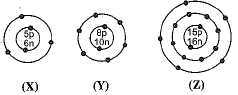Ans.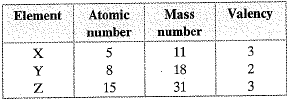Q.10. In response to a question, a student stated that in an atom, the number of protons is greater than the number of neutrons, which in turn is greater than the number of electrons. Do you agree with the statement? Justify your answer.
Ans.
No, it is not possible. Number of protons cannot be greater than number of electrons in case of neutral atoms. These should be equal. Number of protons also cannot be greater than neutrons except in the case of Hydrogen.

Q.11. Calculate the number of neutrons present in the nucleus of an element X which is represented as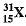Ans.
Element isNo. of Neutrons — Mass number - Atomic number = 31 - 15 = 16

Q.12. Match the names of the Scientists given in column A with their contributions towards the understanding of the atomic structure as given in column B.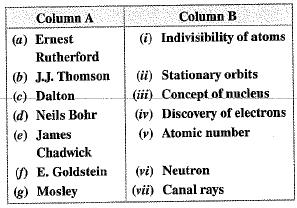Ans.
(a) → iii, (b) → iv, (c) → i, (d) → ii, (e) → vi, (f) → vii, (g) → v

Q.13. The atomic number of calcium and argon are 20 and 18 respectively, but the mass number of both these elements is 40. What is the name given to such a pair of elements?

Ans. They are isobars.

Q.14. Complete the table on the basis of information available in the symbols given below: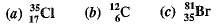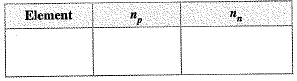Ans.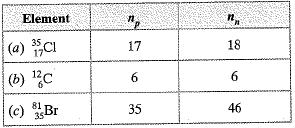Q.15. Helium atom has 2 electrons in its valence shell but its valency is not 2. Explain.
Ans.
Helium atom is zero valent because its first shell is the outermost shell which is full and therefore, it is stable. It cannot gain or lose or share electrons.

Q.16. Fill in the blanks in the following statements:
(a) Rutherford’s α -particle scattering experiment led to the discovery of the ______.
(b) Isotopes have the same  ______ but different ______.
(c) Neon and chlorine have atomic numbers 10 and 17 respectively. Their valencies will be ________and______respectively.
(d) The electronic configuration of silicon is ______and that of sulphur is ______ .

Ans. (a) Atomic nucleus
(b) atomic number, mass number
(c) 0, 1
(d) Silicon - 2, 8, 4; Sulphur - 2, 8, 6.

Q.17. An element X has a mass number 4 and atomic number 2. Write the valency of this element.
Ans. Its atomic number is 2, it has 2 electrons in 1st shell. Its valency is zero as it has stable electronic configuration.

Q.18. Why do Helium, Neon and Argon have zero valency?
Ans.
Helium has 2 electrons in its valence shell whereas Neon and Argon have 8 electrons in their valence shells. They have maximum possible number of electrons in their valence shells, so, they have no tendency to gain or lose or share electrons to form bonds. Therefore, they are zero valent.

Q.19. The ratio of the radii of hydrogen atom and its nucleus is 10: 1. Assuming the atom and the nucleus to be spherical, (a) What will be the ratio of their sizes? (b) If atom is represented by planet earth ‘Re’ = 6.4 x 106 m, estimate the size of the nucleus.
Ans.
(a) Volume of sphere = 4/3πr3
Volume of atom = 4/3πR[where ‘R ’ is radius o f atom]
Volume of nucleus = 4/3πr[where ‘r’ is radius of nucleus]
∴  Volume of atom = 4/3π (105r)
= 4/3πx1015r3
Ratio of the volume of atom to that of nucleus =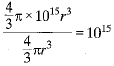(b) If the atom is represented by the planet earth (Re = 6.4 x 106m) then the radius of the nucleus would be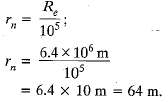Q.20. Enlist the conclusions drawn by Rutherford from his α-ray scattering experiment.
Ans.
(a) Most part of atom is hollow as most of α- rays went undeviated through the gold foil.
(b) Very few α-rays (1%) deviated from their path, so very small portion in the centre of atom is positively charged called nucleus.
(c) A very small number of α-rays came back in the same path showing that size of nucleus is very small and heavy.

Q.21. In what way is the Rutherford’s atomic model different from that of Thomson’s atomic model?
Ans.
According to Rutherford, atom consists of positively charged centre called nucleus. Electrons revolve around the nucleus in fixed path called orbits. The nucleus is very small as compared to atom. The density of nucleus is very high as its size is small and whole mass of atom is concentrated into the nucleus.
According to Thomson, the electrons are embedded in a positively charged sphere like seeds of watermelon and the mass of atom was assumed to be uniformly distributed.

Q.22. Show diagramatically the electron distributions in a sodium atom and a sodium ion and also give their atomic number.
Ans.
Na (11) = 2, 8, 1
Na+ (11) = 2, 8
e- = 11 - 1 = 10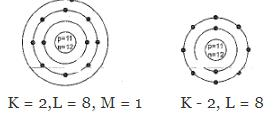Atomic number = 11 Atomic number = 11 Atomic number remains the same as it is equal to number of protons.

Q.23. In the Gold foil experiment of Geiger and Marsden, that paved the way for Rutherford’s model of an atom, ∼ 1.00% of the α-particles were found to deflect at angles > 50°. If one mole of α-particles were bombarded on the gold foil, compute the number of α-particles that would deflect at angles less than 50°.
Ans.
No. of α-particles deviated at angle > 50° = 1 %
No. of α-particle deflected at less than 50° = 100 - 1 = 99%
No. of α-particles bombarded = 6.022 x 1023
No. of α-particles that deflected at an angle less than 50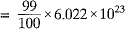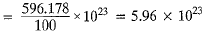Offer running on EduRev: Apply code STAYHOME200 to get INR 200 off on our premium plan EduRev Infinity!

## Science Class 9

95 videos|223 docs|135 tests

,

,

,

,

,

,

,

,

,

,

,

,

,

,

,

,

,

,

,

,

,

;